Part 2 – Who Will Leave?

In Part 1[i], we estimated the probability or the label of the promotion of a given worker in an entity. In this second article, the probability or the label of an employee exit will be measured. This is a crucial measure for all organizations across the world as the tangible and visible estimate of an employee exit will save an organization a lot of time and money.

Not only will we know the probability of the exit of a current employee, but also, we can have a good idea of whether a new employee will leave the organization or not. Although Azerbaijani companies are a long way from implementing data-driven strategies in the near or mid-future, the techniques applied in this paper will be extremely useful for any organization with sound HR data.

For example, if a loan officer at a bank in Azerbaijan leaves that bank for another, most probably they will carry their accumulated loan portfolio to the new bank. This is a huge risk and knowing the probability that a specific loan officer will leave enables the bank to take preliminary actions. The same example can be applied to other situations and job positions.

The methodology applied in this paper is applicable and valuable for companies in Azerbaijan, as well as in other countries. Its application would benefit HR and help develop better working conditions. The value of this methodology is even greater for the Azerbaijani labor market as working conditions are poor and employee loyalty is low.

To expand the applicability of our approach, we do not speculate about the meaning of the features. This is also helpful for Azerbaijani organizations that do not have extensive employee data. Although the abundance of data can make the estimation more precise, our approach estimates the probability of exit with what is available. Thus, our methodology is universal for any organization. As in the first part of the article, we employ statistical learning tools such as penalized logistic regressions (Ridge, Lasso) and machine learning methods such as KNN and SVM.

Data and Methodology

We have more than 12,000 observations with around 6 initial features. The response variable shows whether a worker left the company or not. More features were created out of the initial ones by taking squares or cubes of the suitable continuous ones, but there was no gain in predictive power.

Moreover, the standardization and normalization of variables was tried but there was no gain in predictive power. As a result, variables were used in levels. Normalization was used only for the KNN[ii] estimator as it does not work otherwise.

Around 17% of the data has an exit tag equal to 1. We split the dataset into two parts: 70% for training our models and 30% for testing them. It is split automatically in a way that both parts share the same rate of success (here the exit tag, a worker who has left).

Starting with 11 penalized shrinkage logistic regressions, 11 models were returned by tuning the alpha parameter from 0 to 1 with 0.1 intervals. When alpha equals zero, the regression is called Ridge. When it equals 1, it is called LASSO.  Any value in between would be called elastic-net, especially when alpha is equal to 0.5. Further research could use more alpha parameters with more computing power and time.

These models are executed on the training set and get their AUROC[iii] (simply AUC from now on) for comparison. As is conventional for shrinkage models, we input all features and let the model decide which ones to keep and which ones to shrink to zero (thus insignificant). We need to emphasize that the Ridge regression where the alpha parameter is set to zero keeps all features.

Results for Statistical Learning

First, we will present the results from the penalized logistic regressions. The AUC for these models over the training set will be compared. For parsimony and to save time, we skip the conventional non-penalized logistic regression since we will have to go further with more modern techniques.

Table 1. Results for Penalized Logistic Regressions

 alpha AUC 0 0.852727678189757 0.1 0.851843491786532 0.2 0.851098038353651 0.3 0.85130858403536 0.4 0.851295735120991 0.5 0.850227322390076 0.6 0.850910558042536 0.7 0.850941817777063 0.8 0.850163845304546 0.9 0.850946921836748 1 0.850797344962633

From the table above we highlight the three best models based on their AUC and respective alpha parameter. Two of them can be labeled as elastic-net regressions and one of them as the Ridge (thus 0). We will use them to choose the model with the highest predictive power on the unseen test data.

The best model seems to be the Ridge model with an alpha parameter of 0. This model keeps all features available. In the first part of this article, out of 20 features a sizeable 70% was kept and the Ridge model was not deemed the best. The difference could be due to the availability of information. Each model tries to optimize the combinations but with a limited number of features (6) the models cannot leave out any information.

Although the numbers seem close to each other, the graph below shows how volatile the prediction power can be. It is also known that shrinkage models overcome ill-conditioned cases such as highly correlated variables and quasi-separation problems, resulting in lower prediction error. Note that the Ridge can keep highly correlated variables in the same model. The model will not leave out any information even if we have almost identical variables with negligible difference.

Graph 1. AUCs of Training Set Models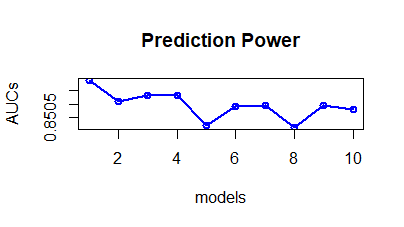The study’s test set includes the following values: 0 and 1. However, the models predict probabilities between zero and one. A function predicting classes could be used but would make the program choose the cutoff value for probabilities as 0.5, which is not justified due to oversimplification. A cutoff value of 0.5 means above 0.5 class is predicted as 1, otherwise as 0.

Although it seems a priori normal, we need to check each dataset for justification of such a cutoff. Here we need to introduce criteria for which we obtain cutoff values and then compare models. We can use the AUC as a test set as well but we need more universal criteria to make the models comparable with machine learning counterparts such as SVM.

Three concepts need to be defined: accuracy, sensitivity, and specificity. Accuracy is the portion that has been captured by the model correctly. If the accuracy is 90%, then 90% of the zeros and ones were predicted correctly. Sensitivity measures the rate at which a model correctly predicts true positive (TP) rates. On the contrary, specificity measures the rate at which a model correctly predicts negatives (0).

Three criteria are used to choose the cutoff values for each of the three models. The first is the cutoff that maximizes accuracy. The second maximizes both sensitivity and specificity, minimizing the distance between the upper left corner of the ROC curve graph and the curve itself.

The last criterion is the cost-minimizing cutoff, which minimizes the self-defined cost function. This cost function sums up false negatives and false positives to achieve a situation where false negatives are twice as costly as false positives. In this case, it means that the cost of mislabeling a leaving worker as staying in the organization is more costly than mislabeling a staying worker as leaving. Let’s look at the numbers of the three selected models to pin them down to one model.

Table 2. Models and Their Performance on the Test Set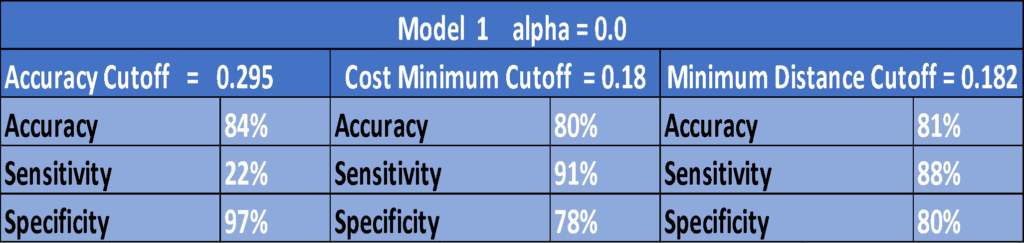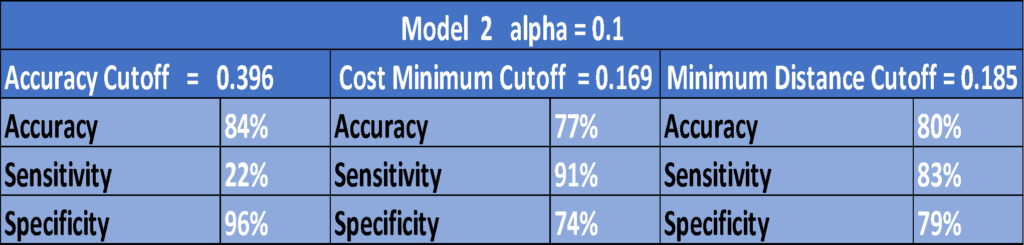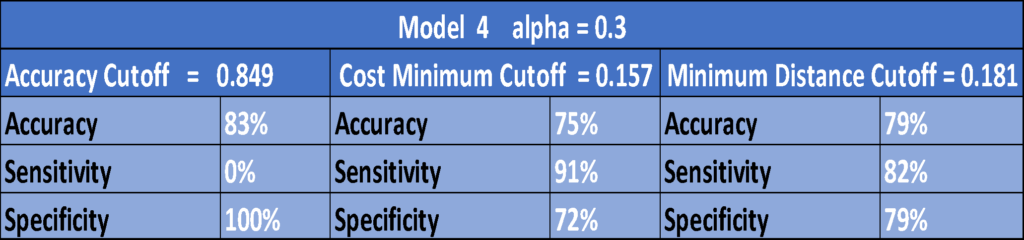Several observations need to be mentioned. First, we observe that accuracy cutoffs give us the highest accuracy, as expected. If, on the other hand, we use a cost minimizer cutoff, we gain more than four times on sensitivity with very little loss on accuracy and specificity.

The third model, surprisingly, returns an accuracy cutoff of 85% with 0 sensitivity and 100% specificity. We wanted exactly the opposite. This means that with this model and this cutoff we cannot predict correctly whether anyone will leave the job. On the columns to the right, we do not observe anything different from the middle ones with cost minimizer cutoffs.

In fact, cutoff values are very close to each other with a cost minimizer and a distant minimizer. It is reassuring that our self-built cost function is quite useful. I would go for a model with the highest sensitivity and specificity with the least possible loss on accuracy. That would be our first model, the Ridge with an alpha parameter which keeps all features and with a cost minimizer cutoff value of 0.18. Note that our cutoff value is less than half of 0.5, which the program would automatically have chosen.  Let’s look at our best model (model 1, the Ridge) more closely.

Graph 2. ROC Curve of the ModelIn the graph above, the hit-rate is sensitivity and fall-out is the false positive rate, which is one minus specificity. Thus, the minimal distance from the upper left corner to the curve maximizes sensitivity and specificity since the uppermost left corner is the ideal point.

Graph 3. Confusion Matrix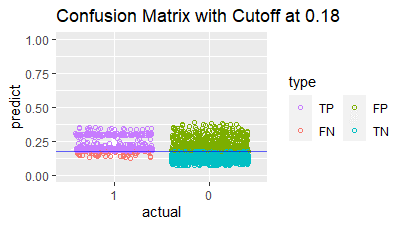Above is shown a confusion matrix of suitable points for each category (True Positive, True Negative, False Positive, False Negative). The number of TPs is quite high since we chose the cutoff with the highest sensitivity (hit-rate).

Graph 4. Predicted Train and Test Scores’ Overlap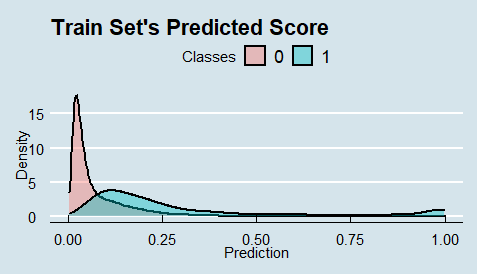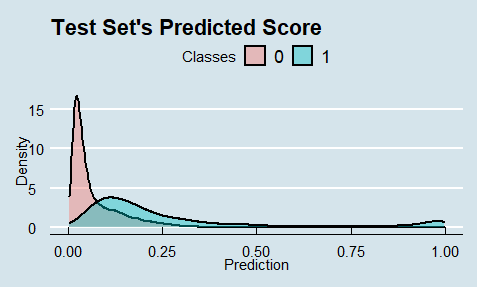The graphs above show the densities of predictions per class. Attention is needed since we observe quite an overlap for the data given. Moreover, the graphs indicate that our cost minimizer cutoff value 0.18 is fairly reasonable.

Machine Learning Results

Several basic machine learning tools have been used for further classifications. Unlike the previous ones, these methods yield classes (0 and 1) as predicted outcomes. Thus, there is no cutoff problem or otherwise decision freedom.

Some of the techniques require much more time and computing power than others. Running those methods on all features might take hours to yield results. Thus, we use the LASSO operator (where alpha is equal to 1) from the previously run models for feature selection. Below are the results of some machine learning techniques.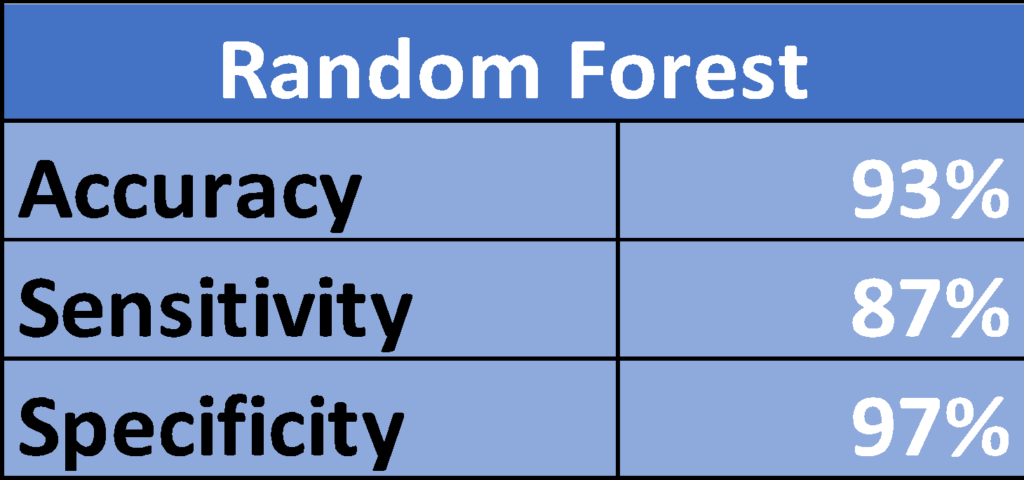There are several points to make about the above results. First of all, we observe that accuracy is highest with a KNN estimator, SVM being the second best. The accuracies of these models are higher than the shrinkage logistic models ran before. This is a very different result from the previously tested HR data.

Looking at the sensitivities, SVM outperforms the machine learning methods presented above and previous shrinkage models. By losing only 1% on the accuracy, we would choose SVM estimator for our employee exit prediction. It is important to note that we need to test and try these models to understand which one to use and why.

Conclusion and Further Discussion

We found that our best method for this data is the Support Vector Machine. In the last article, we also used HR data for a similar purpose and ended up with a different model. Note that in both cases 20 models were used to choose from.

It is recommended to use at least the same models as in this paper or more if possible. Furthermore, each dataset might need a separate approach, but the goal is to return the best prediction with the lowest error.

One achievement of this second paper is that using only statistical learning or machine learning is not enough. We strongly recommend mixing and trying both models to get a more precise result. The use of machine learning methods solely means ignoring otherwise important statistically powerful tools such as logistic regression, which is theoretically nutritious . In the same way, solely using statistical learning methods means ignoring modern, powerful predictive tools such as the Support Vector Machine. It is important to note that LASSO is the borderline for both and has a selective feature.

[i] https://bakuresearchinstitute.org/en/hr-data-analytics-using-statistical-machine-learning/

[ii] KNN- k nearest neighbor

[iii] AUROC – The area under the receiver operating characteristic

Part-1

HR Data Analytics Using Statistical Machine Learning